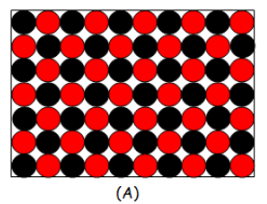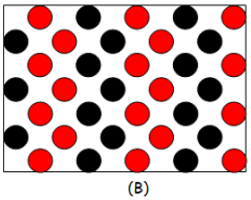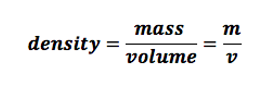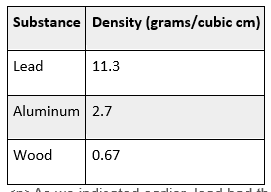# Density: Definition, Formula

#### Video Lesson on Density: Definition, Formula

This lesson video Not available at this time available video coming soon

### Density: Definition, Formula & Practice Problems

In this lesson, you will learn about density, the way in which density can be calculated from mass and volume, and that density of substances are constant. You will get to calculate densities of various materials in different situations.

### Definition

Let's say we have three identical cubes (each side of the cube has a 1.0 centimeter length). One cube is made out of lead, the second cube is made out of aluminum, and the third cube is made out of wood. If I asked you which cube would be the most dense of the three cubes, you will most likely say the one made out of lead. Simple enough. But just what exactly is density? Density is defined as the ratio between mass and volume or mass per unit volume. It is a measure of how much stuff an object has in a unit volume (cubic meters or cubic centimeters). Mass is the measure of how much stuff an object contains and volume is the measure of how much space an object occupies in three-dimensional space. The figure below shows a dense object versus a less dense object.Dense versus Less Dense

As you can see in the figure, the dense object (A) has more stuff than the less dense object (B). Both objects are shown to occupy the same space.

### Calculating Density

So, how do we find the density of an object? As you saw in the definition, you need two pieces of information in order to calculate density: mass (in grams or kilograms), and volume (in cubic centimeter or cubic meter). The formula for density is:Going back to the three cubes in the beginning of the lesson, let us calculate the densities of the three objects. Let's say we have 11.3 grams of lead, 2.7 grams of aluminum, and 0.67 grams of wood (pinewood). The densities are calculated as mass per unit volume (1 cubic centimeter).As we indicated earlier, lead had the highest density of the three objects with the same volume. We also point out that no matter what volume of lead, aluminum, or wood you have, the densities of all three objects will always be the same. Densities of substances do not change. Once you know the density of lead, that density will never change. So, let's say you have an object made up of an unknown substance. You measure the mass of the object and the volume of the object and get 11.3 grams per cubic centimeter. Then that unknown substance is lead!

### Practice Problems

What else is unknown at this point? How well you've understood the concept of density! It may seem easy but let's just make sure that's really the case with a bunch of good old practice problems.

Let's start with some easy ones before moving to harder ones.

### Question 1

Q1. The mass of object X is 10 grams (g) and its volume is 1 cubic centimeter (cc). What is its density?

A1. This one is super easy. We have density (d) = m/v, so d = 10/1 = 10 g/cc.

### Question 2

Q2. It's 2050 and Ethorium is a new alien substance recently discovered by the Mars One mission. It has a mass of 1500 g in a volume of 100 cc. What is its density?

A2. d = 1500/100 = 15 g/cc.

### Question 3

Q3. What is the name of the element that has 42.9 grams of matter per 2 cubic centimeters?

A3. This would require some calculation and just a tad of research using the internet. We set d = m/v to d = 42.9/2 = 21.45 g/cc. Looking up this density online we see that it refers to platinum.

### Question 4

Q4. All else equal, substance Z expands with increasing temperature. What can you say about its density as the temperature increases?

A4. The density will decrease. This is because mass stays the same but volume increases. In d = m/v, the denominator increases while the numerator stays the same. That means d gets smaller.

### Question 5

Q5. Albert Edison is a famous scientist who recently figured out that the density of a new substance he created is 76 g/cc. He remembers that the mass was 7.6 g but can't, for the life of him, recall what volume of space the substance occupied when he figured that out. Help him out!

A5. We know that d = 76 g/cc. So now we set that equal to our mass divided by our unknown volume (x). In other words:

76 = 7.6/x

Next, we need to re-arrange the equation so that x is left on one side:

7.6/76 = x

Solve to get x = 0.10. In other words, the volume was 0.10 cubic centimeters. Double check by writing d = m/v, then filling in the mass and volume to get d = 7.6/0.1 = 76.

### Question 6

Q6. What is the density of a substance with a mass of 27 g found in a cube that has a length of 3 cm?

A6. A cube is a 3D object where the length, width, and height are all the same and a volume of a cube is its length x width x height. This means the volume here is 3 x 3 x 3 = 27 cc.

Therefore d = 27/27 = 1 g/cc; which happens to be the density of water (at 4 degrees Celsius).

Question 7

Q7. You are a pirate. You've found a secret pirate map where X marks the spot. You know there is treasure to be found. You set sail, reach shore, dig, and find a treasure chest. You open it up and you see what looks like a large gold rectangular bar with its mass stamped right on top. It reads 5000 g. Your find that the bar measures 20 cm x 10 cm x 5 cm.

You can't believe your luck. Everyone is happy. But, old Sour Pegleg, the pirate no one likes, is a bit sour on this one. He thinks its fool's gold cubes, or iron pyrite cubes. The other pirates disagree. They can smell real gold from a mile away. Sour Pegleg, pessimist he is, is quite smart though. He knows that the density of fool's gold is about 5 g/cc while the density of real gold is about 19 g/cc. Given this, how can we tell who is right?

A7. First, we need to know what the volume is of this bar. A rectangular prism has a volume of length x width x height or 20 cm x 10 cm x 5 cm in this case. This is a volume of 1000 cc. So d = 5000/1000 = 5 cc. Despite this huge'gold' bar, old Sour Pegleg was right to be suspicious: it's just fool's gold.

### Lesson Summary

Density gives you information about how much stuff is in an object divided by how much space the object occupies. So, you can measure the mass of an object (in grams or kilograms), measure how much space the object occupies in three-dimensional space (in cubic centimeters or cubic meters), then divide the mass by the volume to get the density of the object. Once you know the density of a substance (like lead, aluminum, or pinewood), that density will not change even if you pick a larger or smaller volume than the volume you used to make your initial calculation. Densities of substances are constant.

kkk

### Next Topics